Definitions of Square Dance Calls and Concepts
1/4 (or 3/4) Wheel The Ocean | Sea [C3A]

Index -->  Plus  |  A1  |  A2  |  C1  |  C2  |  C3A  |  C3B  |  C4  |  NOL  |
Definitions (Text Only) -->  Plus  |  A1  |  A2  |  C1  |  C2  |  C3A  |  C3B  |  C4  |  NOL  |
 Find call:

1/4 (or 3/4) Wheel The Ocean | Sea -- [C3A]
(Bob Urbasik 1984)

 \$B8@8l(B1/4 Wheel The Ocean | Sea:
\$B:8\$N(B Two-Faced Line \$B\$+\$i!%(BLeft Cast 1/4 \$B\$r\$7(B (\$B8~\$+\$\$9g\$C\$?%+%C%W%k\$H\$J\$j(B), Finish Wheel The Ocean | Sea \$B\$r\$7\$^\$9!%(B(Ocean: Belles \$B\$,

3/4 Wheel The Ocean | Sea:
\$B1&\$N(B Two-Faced Line \$B\$+\$i!%(BLeft Cast 3/4 \$B\$r\$7(B (\$B8~\$+\$\$9g\$C\$?%+%C%W%k\$H\$J\$j(B), Finish Wheel The Ocean | Sea \$B\$r\$7\$^\$9!%(BMini-Wave \$B\$N(B Box \$B\$G=*\$o\$j\$^\$9!%(B

1/4 (\$B\$^\$?\$O(B 3/4) Wheel The Ocean | Sea \$B\$O(B 2 \$B%Q!<%H\$N%3!<%k\$G\$9!%(B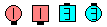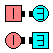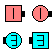1/4 Wheel The Ocean\$B\$NA0(B Left Cast 1/4\$B\$N8e(B Belles diagonalRight Pull By\$B\$N8e(B (\$B=*\$o\$j(B)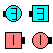1/4 Wheel The Sea\$B\$NA0(B Left Cast 1/4\$B\$N8e(B Belles Walk, Beaus Dodge\$B\$N8e(B (\$B=*\$o\$j(B)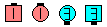3/4 Wheel The Sea\$B\$NA0(B Left Cast 3/4\$B\$N8e(B Belles Walk, Beaus Dodge\$B\$N8e(B (\$B=*\$o\$j(B)

\$BCm
• 1/4 (\$B\$^\$?\$O(B 3/4) Wheel The Ocean | Sea \$B\$O(B, \$B;O\$a\$N(B Left Cast (Wheel Around) \$B\$,(B 1/2 \$B\$G\$J\$/(B 1/4 (\$B\$^\$?\$O(B 3/4) \$B\$H\$\$\$&\$3\$H\$r=|\$-(B, Wheel The Ocean | Sea [C2] \$B\$HF1\$8\$G\$9!%(B
• Wheel The Ocean | Sea \$B\$H0c\$C\$F(B, \$BA40w\$,(B (Trailers \$B\$b(B) \$B%3!<%k\$N;O\$a\$N%Q!<%H\$r\$7\$^\$9!%(B
• Cast \$B\$O>o\$K(B Left \$B\$G\$9!%(B
• Wheel The Ocean | Sea \$B\$HF1\$8\$G(B, Belles \$B\$,>o\$KH?BPB&\$X9T\$/?M\$K\$J\$j\$^\$9!%(B
• Wheel The Ocean [C2] (Betty Fricker 1972): \$BGXCf9g\$o\$;\$N%+%C%W%k\$+(B Tandem \$B\$N%+%C%W%k\$+\$i!%(BLeads (\$B\$^\$?\$O;X<(\$5\$l\$??M(B) \$B\$,(B Wheel Around \$B\$r\$7(B, Belles \$B\$OWheel The Sea [C2] (Betty Fricker 1972): \$BGXCf9g\$o\$;\$N%+%C%W%k\$+(B Tandem \$B\$N%+%C%W%k\$+\$i!%(BLeads (\$B\$^\$?\$O;X<(\$5\$l\$??M(B) \$B\$,(B Wheel Around \$B\$r\$7(B, Belle Walk Beau Dodge \$B\$r\$7\$^\$9!%:8\$N(B Mini-Wave \$B\$N(B Box \$B\$G=*\$o\$j\$^\$9!%(BChoreography for 1/4 (or 3/4) Wheel The Ocean | SeaComments? Questions? Suggestions?

https://www.ceder.net/def/14wheeltheocean.php?level=master&language=japan
11-December-2019 14:17:14